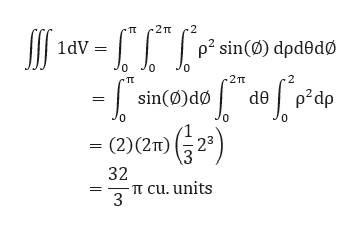# Use geometry to evaluate ∫∫∫R 1 dV where R is the region inside the sphere x2+y2+z2=4.

Question
33 views

Use geometry to evaluate ∫∫∫1 dV where R is the region inside the sphere x2+y2+z2=4.

check_circle

Step 1

Given R as the region inside the sphere

Step 2

Since the given geometric region is the region inside of a sphere, we use the spherical coordinate.

Step 3

And we get a vo...help_outlineImage Transcriptionclose2TT 2 TT p2 sin(Ø) dpdedø 1dV 2TT 2 TT p2dp sin(ø)dø de (2)(2m) 2 32 Tt cu. units 3 fullscreen

### Want to see the full answer?

See Solution

#### Want to see this answer and more?

Solutions are written by subject experts who are available 24/7. Questions are typically answered within 1 hour.*

See Solution
*Response times may vary by subject and question.
Tagged in

### Calculus